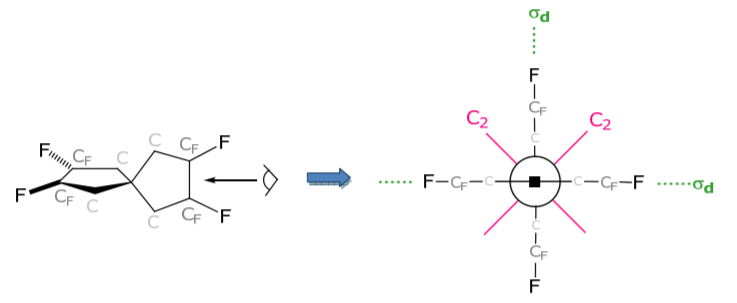# 1.4: Molecular Point Groups 1

$$\newcommand{\vecs}{\overset { \rightharpoonup} {\mathbf{#1}} }$$ $$\newcommand{\vecd}{\overset{-\!-\!\rightharpoonup}{\vphantom{a}\smash {#1}}}$$$$\newcommand{\id}{\mathrm{id}}$$ $$\newcommand{\Span}{\mathrm{span}}$$ $$\newcommand{\kernel}{\mathrm{null}\,}$$ $$\newcommand{\range}{\mathrm{range}\,}$$ $$\newcommand{\RealPart}{\mathrm{Re}}$$ $$\newcommand{\ImaginaryPart}{\mathrm{Im}}$$ $$\newcommand{\Argument}{\mathrm{Arg}}$$ $$\newcommand{\norm}{\| #1 \|}$$ $$\newcommand{\inner}{\langle #1, #2 \rangle}$$ $$\newcommand{\Span}{\mathrm{span}}$$ $$\newcommand{\id}{\mathrm{id}}$$ $$\newcommand{\Span}{\mathrm{span}}$$ $$\newcommand{\kernel}{\mathrm{null}\,}$$ $$\newcommand{\range}{\mathrm{range}\,}$$ $$\newcommand{\RealPart}{\mathrm{Re}}$$ $$\newcommand{\ImaginaryPart}{\mathrm{Im}}$$ $$\newcommand{\Argument}{\mathrm{Arg}}$$ $$\newcommand{\norm}{\| #1 \|}$$ $$\newcommand{\inner}{\langle #1, #2 \rangle}$$ $$\newcommand{\Span}{\mathrm{span}}$$$$\newcommand{\AA}{\unicode[.8,0]{x212B}}$$

The symmetry properties of molecules (i.e. the atoms of a molecule form a basis set) are described by point groups, since all the symmetry elements in a molecule will intersect at a common point, which is not shifted by any of the symmetry operations. There are also symmetry groups, called space groups, which contain operators involving translational motion.

The point groups are listed below along with their distinguishing symmetry elements

C1 : E (h = 1) $$\Longrightarrow$$ no symmetry

Cs : σ (h = 2) $$\Longrightarrow$$ only a mirror planeCi : i (h = 2) $$\Longrightarrow$$ only an inversion center (rare point group)isomer of dichloro(difluoro)ethane

Cn : Cn and all powers up to Cn n = E (h = 2) $$\Longrightarrow$$ a cyclic point groupCnv : Cn and nσv (h = 2n) … by convention a σv contains Cn (as opposed to σh which is normal to Cn). For n even, there are $$\frac{n}{2} \sigma_{v}$$ and $$\frac{n}{2} \sigma_{v_{v}}$$ ' with the σv containing the most atoms and the σvs containing the least atomsConsider a second example:Cnh : Cn and σh (normal to Cn) are generators of Sn operations as well (h = 2n)S2n : S2n and all powers up to S2n 2n = E (h = 2n).The F’s do not lie in the plane of the cyclopentane rings. If they did, then other symmetry operations arise; these are easiest to see by looking down the line indicated below:Note Sn, where n is odd, is redundant with Cnh because Sn n = σh for n odd. As an example consider a S3 point group. S3 is the generator for S3, S3 2 (= C3 2 ) S3 3 (= σh), S3 4 (= C3), S3 5 , S3 6 (= E). The C3’s and σh are the distinguishing elements of the C3h point group.

This page titled 1.4: Molecular Point Groups 1 is shared under a CC BY-NC-SA 4.0 license and was authored, remixed, and/or curated by Daniel Nocera via source content that was edited to the style and standards of the LibreTexts platform; a detailed edit history is available upon request.